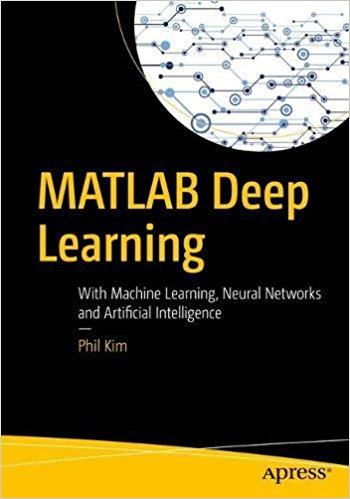# MATLAB Deep Learning – pdf – 电子书免费下载Get started with MATLAB for deep learning and AI with this in-depth primer. In this book, you start with machine learning fundamentals, then move on to neural networks, deep learning, and then convolutional neural networks. In a blend of fundamentals and applications, MATLAB Deep Learning employs MATLAB as the underlying programming language and tool for the examples and case studies in this book.

With this book, you’ll be able to tackle some of today’s real world big data, smart bots, and other complex data problems. You’ll see how deep learning is a complex and more intelligent aspect of machine learning for modern smart data analysis and usage.

What You’ll Learn

• Use MATLAB for deep learning
• Discover neural networks and multi-layer neural networks
• Work with convolution and pooling layers
• Build a MNIST example with these layers

Who This Book Is For

Those who want to learn deep learning using MATLAB. Some MATLAB experience may be useful.

ISBN:1484228448

## 锦瑟无端五十弦，一弦一柱思华年

### 觉得文章有用就打赏一下文章作者

#### 支付宝扫一扫打赏#### 微信扫一扫打赏/* */